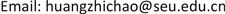HJWCHans Journal of Wireless Communications2163-3983Scientific Research Publishing10.12677/HJWC.2018.82005HJWC-24487HJWC20180200000_51297184.pdf信息通讯 一种基于PSS序列的OFDM系统低复杂高精度帧同步方法及其FPGA实现 A Low Complex and High Precision Frame Synchronization Method for OFDM System Based on PSS Sequence and Its FPGA Implementation 志超1*12东南大学信息科学与工程学院，江苏 南京null* E-mail:huangzhichao@seu.edu.cn(黄志);2404201808024556© Copyright 2014 by authors and Scientific Research Publishing Inc. 2014This work is licensed under the Creative Commons Attribution International License (CC BY). http://creativecommons.org/licenses/by/4.0/

OFDM，帧同步，低复杂度，PSS，LabVIEW FPGA, OFDM Frame Synchronization Low Complex PSS LabVIEW FPGA1. 引言

2. 低复杂高精度帧同步算法

2.1. LTE中同步序列PSS

PSS序列是由长度为63点的ZC  序列(Zadoff-Chu)组成，本身为频域信号，具有自相关性极高互相关性极低的特点，通过IFFT (Inverse Fast Fourier Transformation)转换到时域，所得时域信号也具有同样的特点，适用于加入到无线帧的头部，可用于无线帧同步使用。

a q = exp [ − j 2 π q n ( n + 1 ) / 2 + l * n N Z C ] (1)

ZC序列有以下特性：

1) ZC序列具有恒定振幅，经过IFFT也是恒定振幅的。这限制了峰均值比，降低了对数据信号的干扰。

2) 任何长度的ZC序列具有极好的循环自相关性。

Z C q 63 ( n ) = exp [ − j π q n ( n + 1 ) 63 ] (2)

2.2. 粗同步算法

2.3. 高精度帧同步算法

3. 高精度无线帧同步的LabVIEW FPGA实现

LabVIEW FPGA是美国国家仪器公司推出的一款图形化界面FPGA开发语言   ，具有开发简单、可读性强等特点，被广泛应用于该公司的FPGA系列芯片的开发中，本论文采用该语言进行帧同步算法的实现。粗同步模块是高精度无线帧同步算法的必须步骤，首先简要介绍粗同步模块的实现    。

C n = ∑ i = 1 128 ( r e l o c a l , i + i ∗ i m l o c a l , i ) ( r e t i m e , n + i + i ∗ i m t i m e , n + i ) ∗ = ∑ i = 1 128 [ ( r e l o c a l , i ∗ r e t i m e , n + i + i m l o c a l , i ∗ i m t i m e , n + i ) + i ( − r e l o c a l , i ∗ i m t i m e , n + i + i m l o c a l , i ∗ r e t i m e , n + i ) ] = ∑ i = 1 128 r e l o c a l , i ∗ r e t i m e , n + i − i ∗ ∑ i = 1 128 r e l o c a l , i ∗ i m t i m e , n + i + i ∗ ∑ i = 1 128 i m l o c a l , i ∗ r e t i m e , n + i + ∑ i = 1 128 i m l o c a l , i ∗ i m t i m e , n + i (3)

4. 应用案例

5. 结论